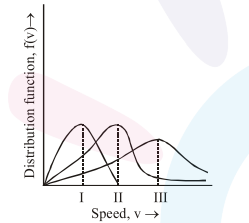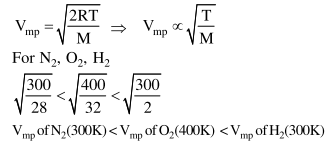# Points I, II and III in the following plot respectively correspond to

Question:

Points I, II and III in the following plot respectively correspond to

$\left(\mathrm{V}_{\mathrm{mp}}\right.$ : most probable velocity)1. $\mathrm{V}_{\mathrm{mp}}$ of $\mathrm{N}_{2}(300 \mathrm{~K}) ; \mathrm{V}_{\mathrm{mp}}$ of $\mathrm{H}_{2}(300 \mathrm{~K})$;

$\mathrm{V}_{\mathrm{mn}}$ of $\mathrm{O}_{2}(400 \mathrm{~K})$

2. $\mathrm{V}_{\mathrm{mp}}$ of $\mathrm{H}_{2}(300 \mathrm{~K}) ; \mathrm{V}_{\mathrm{mp}}$ of $\mathrm{N}_{2}(300 \mathrm{~K})$

$\mathrm{V}_{\mathrm{mp}}$ of $\mathrm{O}_{2}(400 \mathrm{~K})$

3. $\mathrm{V}_{\mathrm{mp}}$ of $\mathrm{O}_{2}(400 \mathrm{~K}) ; \mathrm{V}_{\mathrm{mp}}$ of $\mathrm{N}_{2}(300 \mathrm{~K})$

$\mathrm{V}_{\mathrm{mp}}$ of $\mathrm{H}_{2}(300 \mathrm{~K})$

4. $\mathrm{V}_{\mathrm{mp}}$ of $\mathrm{N}_{2}(300 \mathrm{~K}) ; \mathrm{V}_{\mathrm{mp}}$ of $\mathrm{O}_{2}(400 \mathrm{~K})$;

$\mathrm{V}_{\mathrm{mp}}$ of $\mathrm{H}_{2}(300 \mathrm{~K})$

Correct Option: , 4

Solution: